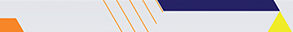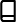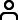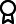Journal metrics
Acceptance rate15%
Submission to final decision42 days
Acceptance to publication64 days
CiteScore1.900
Journal Citation Indicator-
Impact Factor-

### Article of the Year 2020

Mathematical Modelling and Analysis of Transmission Dynamics of Lassa Fever

####Journal profile

Journal of Applied Mathematics publishes original research papers and review articles in all areas of applied, computational, and industrial mathematics.

####Editor spotlight

Chief Editor, Professor Theodore E. Simos, is based at Ulyanovsk State Technical University, Russia. His main research interest is the numerical analysis of differential equations.

####Special Issues

Do you think there is an emerging area of research that really needs to be highlighted? Or an existing research area that has been overlooked or would benefit from deeper investigation? Raise the profile of a research area by leading a Special Issue.

## Latest Articles

More articles
Research Article

### Coordinate Descent-Based Sparse Nonnegative Matrix Factorization for Robust Cancer-Class Discovery and Microarray Data Analysis

Determining the number of clusters in high-dimensional real-life datasets and interpreting the final outcome are among the challenging problems in data science. Discovering the number of classes in cancer and microarray data plays a vital role in the treatment and diagnosis of cancers and other related diseases. Nonnegative matrix factorization (NMF) plays a paramount role as an efficient data exploratory tool for extracting basis features inherent in massive data. Some algorithms which are based on incorporating sparsity constraints in the nonconvex NMF optimization problem are applied in the past for analyzing microarray datasets. However, to the best of our knowledge, none of these algorithms use block coordinate descent method which is known for providing closed form solutions. In this paper, we apply an algorithm developed based on columnwise partitioning and rank-one matrix approximation. We test this algorithm on two well-known cancer datasets: leukemia and multiple myeloma. The numerical results indicate that the proposed algorithm performs significantly better than related state-of-the-art methods. In particular, it is shown that this method is capable of robust clustering and discovering larger cancer classes in which the cluster splits are stable.

Research Article

### A Subsidy Strategy to Boost the Activity of Small Milk Producers in Morocco

The dairy sector in Morocco is a sector that struggles to develop by facing several difficulties. Therefore, a significant gap has emerged between the main national producers and small farmers, while in the economy, the development of small economic actors presents an important lever of growth. If we assume that the consumer’s milk demand function is linear, that it depends only on the consumer’s price, and that production costs follow a decreasing return, and finally, by considering only two actors representing the different types of producers, we will show in this paper that a competitive production subsidy of 0.5 MAD can triple the market share of small farmers while being in a situation of Nash-Cournot’s equilibrium. In addition, the addition of a subsidy to production costs will reduce the price of milk and, thus, increase the annual milk consumption of Moroccans from 59 liters, which is below the world average consumption, to 94 liters annually, thus benefiting the consumer in addition to small farmers.

Research Article

### Approximate Solution of a Reduced-Type Index- Hessenberg Differential-Algebraic Control System

This study focuses on developing an efficient and easily implemented novel technique to solve the index- Hessenberg differential-algebraic equation (DAE) with input control. The implicit function theorem is first applied to solve the algebraic constraints of having unknown state differential variables to form a reduced state-space representation of an ordinary differential (control) system defined on smooth manifold with consistent initial conditions. The variational formulation is then developed for the reduced problem. A solution of the reduced problem is proven to be the critical point of the variational formulation, and the critical points of the variational formulation are the solutions of the reduced problem on the manifold. The approximate analytical solution of the equivalent variational formulation is represented as a finite number of basis functions with unknown parameters on a suitable separable Hilbert setting solution space. The unknown coefficients of the solution are obtained by solving a linear algebraic system. The different index problems of linear Hessenberg differential-algebraic control systems are approximately solved using this approach with comparisons. The numerical results reveal the good efficiency and accuracy of the proposed method. This technique is applicable for a large number of applications like linear quadratic optima, control problems, and constrained mechanical systems.

Research Article

### Viscous Dissipation Effect in the Free Convection of Non-Newtonian Fluid with Heat Generation or Absorption Effect on the Vertical Wavy Surface

The present article analyzes the effect of viscous dissipations on natural convection heat transfer. The power law model for non-Newtonian fluid with heat generation or absorption effect along a sinusoidal wavy surface with isothermal boundary condition is investigated. A simple coordinate transform is employed to map the wavy surface into a flat surface, and also, the fully implicit finite difference method is incorporated for the numerical solution. The findings of this study can help better understand the effect of parameters such as the Brinkman number, heat generation/absorption, wave amplitude magnitude, and generalized Prandtl number on convective heat transfer in dilatant and pseudoplastic non-Newtonian. Results show that as the Brinkman number increases, the amount of heat transfer decreases. This is physically justifiable considering that the fluid becomes warmer due to the viscous dissipation, decreasing its temperature difference with the constant temperature surface. Also, the effect of the power law viscosity index is surveyed. It is demonstrated that the magnitude of the local Nusselt number in the plane leading edge has the smallest quantity for pseudoplastic fluids compared to dilatant Newtonian fluids. Additionally, as the distance from the plane leading edge increases, the heat transfer declines.

Research Article

### Solutions of Two-Dimensional Nonlinear Sine-Gordon Equation via Triple Laplace Transform Coupled with Iterative Method

This article presents triple Laplace transform coupled with iterative method to obtain the exact solution of two-dimensional nonlinear sine-Gordon equation (NLSGE) subject to the appropriate initial and boundary conditions. The noise term in this equation is vanished by successive iterative method. The proposed technique has the advantage of producing exact solution, and it is easily applied to the given problems analytically. Four test problems from mathematical physics are taken to show the accuracy, convergence, and the efficiency of the proposed method. Furthermore, the results indicate that the introduced method is promising for solving other type systems of NLPDEs.

Research Article

### An Analysis of Asymptotic Properties and Error Control under the Exponential Jump-Diffusion Model for American Option Pricing

Our work is aimed at modeling the American option price by combining the dynamic programming and the optimal stopping time under two asset price models. In doing so, we attempt to control the theoretical error and illustrate the asymptotic characteristics of each model; thus, using a numerical illustration of the convergence of the option price to an equilibrium price, we can notice its behavior when the number of paths tends to be a large number; therefore, we construct a simple estimator on each slice of the number of paths according to an upper and lower bound to control our error. Finally, to highlight our approach, we test it on different asset pricing models, in particular, the exponential Lévy model compared to the simple Black and Scholes model, and we will show how the latter outperforms the former in the real market (Microsoft “MSFT” put option as an example).Journal metrics
Acceptance rate15%
Submission to final decision42 days
Acceptance to publication64 days
CiteScore1.900
Journal Citation Indicator-
Impact Factor-Author guidelinesEditorial boardDatabases and indexingAuthor guidelinesEditorial boardDatabases and indexing

Article of the Year Award: Outstanding research contributions of 2020, as selected by our Chief Editors. Read the winning articles.